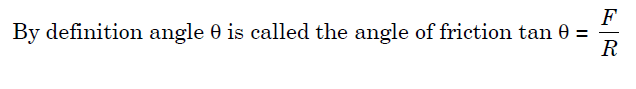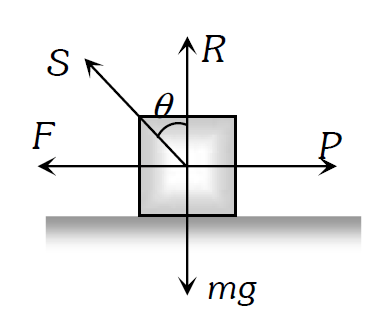# Friction

### Types of Friction

#### (1) Static friction: The opposing force that comes into play when objects are at rest.       (i) In this case static friction F = P.      (ii) Static friction is a self-adjusting force because it changes itself in accordance with the applied force.###### Angle of Friction.

#### Angle of friction may be defined as the angle which the resultant of limiting friction and normal reaction makes with the normal reaction.### Angle of Repose

#### α=θ i.e. angle of repose = angle of friction.

CBSE Class 11 Physics, JEE Physics